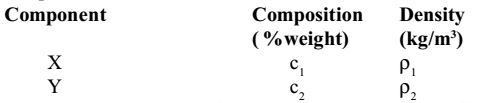## Municipal solid waste miscellaneous

#### Municipal Solid Waste

Direction: A completely mixed activated sludge process is used to treat a wastewater flow of 1 million litres per day (1 MLD) having a BOD5 of 200 mg/l. The biomass concentration in the aeration tank is 2000 mg/l and the concentration of the net biomass leaving the system is 50 mg/l. The aeration tank has a volume of 200 m³.

1. What is the average time for which the biomass stays in the system?

1. The time spend by biomass in system is called sludge age (Qc).

 Qc = Mass of biomass in the system Mass of biomass leaving the system

 = 200 × 10³ × 2000 = 8 days 50 × 106

##### Correct Option: D

The time spend by biomass in system is called sludge age (Qc).

 Qc = Mass of biomass in the system Mass of biomass leaving the system

 = 200 × 10³ × 2000 = 8 days 50 × 106

1. What is the hydraulic retention time of the waste water in aeration tank?

1.  HRT = Volume of tank Inflow

 = 200 × 10³ = 0.2 day 1 × 106 per day

= .2 × 24 = 4.8 hours.

##### Correct Option: B

 HRT = Volume of tank Inflow

 = 200 × 10³ = 0.2 day 1 × 106 per day

= .2 × 24 = 4.8 hours.

Direction: In a rapid sand filter, the time for reaching particle breakthrough (TB) is defined as the time elapsed from start of filter run to the time at which the turbidity of the effluent from the filter is greater than 2.5 NTU. The time for reaching terminal head loss (TH) is defined as the time elapsed from the start of the filter run to the time when head loss across the filter is greater than 3m.

1. The effect of increasing the filter loading rate (while keeping all other conditions same) on TB and TH is

1. TH decreases as head loss is increased with increase in load. TB increases as more time is required to reach break through.

##### Correct Option: A

TH decreases as head loss is increased with increase in load. TB increases as more time is required to reach break through.

1. The effect of increasing the filter depth (while keeping all other conditions same) on TB and TH is

1. When filter depth increases, velocity decreases. Therefore, TH increases. Since the area in bed increases, time to reach break through TB decreases.

##### Correct Option: C

When filter depth increases, velocity decreases. Therefore, TH increases. Since the area in bed increases, time to reach break through TB decreases.

1. Solid waste generated from an industry contains only two components, X and Y as shown in the table belowAssuming (c1 + c2) = 100, the composite density of the solid waste(ρ) is given by:

1. Composite density, ρ

 ⇒ c1 + c2 = c1 = c2 ρ ρ1 ρ2

 100 = c1 = c2 ρ ρ1 ρ2

 ∴ ρ = 100c1 + c2ρ1 ρ2

##### Correct Option: A

Composite density, ρ

 ⇒ c1 + c2 = c1 = c2 ρ ρ1 ρ2

 100 = c1 = c2 ρ ρ1 ρ2

 ∴ ρ = 100c1 + c2ρ1 ρ2